So far, we have discussed Ratios. In this article, let us investigate the basics of Proportion.

Definition:

When two ratios are equal,then the ratios are called proportional, that is, when,then a,b,c, and d are said to be in proportion.

This is also represented as a: b :: c: d and read as a is to b is as c is to d .

In the relation given above:

• a and d are labeled as extremes.
• b and c are labeled as means.

Important Properties for Proportion:

1. Product of extremes = product of means

What does this mean?

If2. Inverse proportion or Invertendo

The following is called an inverse proportion or invertendo:

If3. Componendo and Dividendo

If, then

(i)(Componendo)

How can we prove this result?

Add 1 to each side of the given ratio and we will have this result.(ii)(Dividendo)

How can we prove this result?

Subtract 1 from each side of the given ratio and we will have this result.(iii)(Componendo and Dividendo)

Proof:We have(iv)We have4. Continued Proportion

3 terms or quantities of the same kind are said to be in continued proportion when the ratio of the 1st to the 2nd is equal to the ratio of the 2nd to the 3rd.

Effectively, this means:Here, the second quantity (b) is called the mean proportional between the first (a) and the third (c).

The third quantity (c) is called the third proportional to the first and second.

5. Proportion involving more than 4 terms

If…. then, this can be written asProof:

Let us assume,=…….=k

a =bk,c=dk,e=fk=…… … (1)

Now if the resultto be true, equation 1 need to satisfy it.=1

So=1.

To get a firm hold on the above discussed concepts, test yourself on the exercise below:

Exercise:

Question 1: If p: q = r: s = t: u = 2: 3, then (mp + nr + ot): (mq + ns + ou) is equal to:

(1) 1: 3

(2) 1: 2

(3) 2: 3

(4) 3: 2

Solution: (3)

Ifthen each of these ratios is equal toHere,Multiply p and q by m; r and s by n; t and u by o.By using the formulaQuestion 2: If a: b = c: d = e: f = 1: 2, then (pa + qc + re): (pb + qd + rf) is equal to:

(1) p: (q + r)

(2) (p + q): r

(3) 2: 3

(4) 1: 2

Solution: (4)

Ifthen each of these ratios is equal toNowMultiply a and b by p; c and d by q; e and f by r.By using the formulaQuestion 3: If x: y = 3: 1, then x3 – y3: x3 + y3 =?

(1) 13: 14

(2) 14: 13

(3) 10: 11

(4) 11: 10

Solution: (1)IfThen by componendo and dividendoQuestion 4: The fourth proportional to 0.12, 0.21, 8 is:

(1) 8.9

(2) 16

(3) 14

(4) 17

Solution: (3)

Let the fourth proportional be m.

Then,or m = 8×or m = 8×or m=14

Question 5: If b is the mean proportional of a and c, then (a – b)3: (b – c)3 equals?

(1) a3: c3

(2) b2: c2

(3) a2: c2

(4) a3: b3

Solution: (4)

As per question b is the mean proportional of a and c.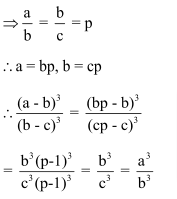Question 6: If a: b = c: d = e: f = 1: 2, then (3a + 5c +7e): (3b + 5d + 7f) is equal to:

(1) 8: 7

(2) 2: 1

(3) 1: 4

(4) 1: 2

Solution: (4)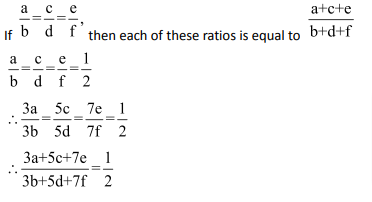Question 7: If a: (b + c) = 1: 3 and c: (a + b) = 5: 7, then b: (a + c) is equal to?

(1) 1: 2

(2) 2: 3

(3) 1: 3

(4) 2: 1

Solution: (1)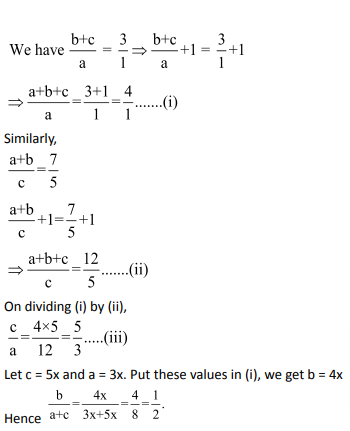Question 8: The mean proportional between (3 + √2) and (12 –√32) is?

(1)(2)(3)6

(4)Solution: (2)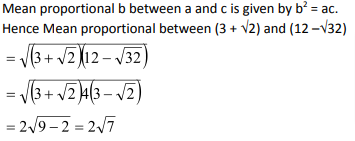Question 9: If a: b = c: d, thenis not equal to?

(1)(2)(3)(4)Solution: (4)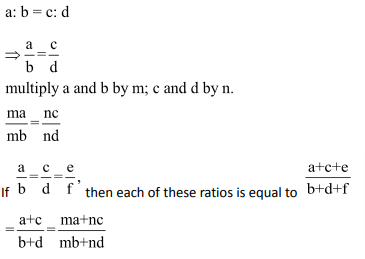Question 10: If A: B = 3: 4 and B: C = 6: 5, then A: (A + C) is equal to

(1)9: 10

(2) 10: 9

(3) 9: 19

(4) 19: 9

Solution: (3)Question 11: If x: y = 5: 6, then (3x2 – 2y2): (y2 – x2) is?

(1) 7: 6

(2) 11: 3

(3) 3: 11

(4) 6: 7

Solution: (3)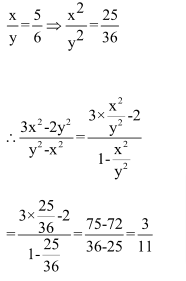Question 12: If x: y = 3: 4, then 4x + 5y: 5x – 2y is?

(1) 7: 32

(2) 32: 7

(3) 4: 3

(4) 5: 2

Solution: (2)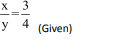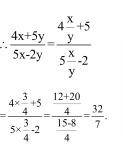### Ratio and Proportion Questions: Problems on Ratio and Proportion you should solve for competitive examination preparation

Welcome to this exercise on Problems on Ratio and Proportion. In this exercise, we build on the basic concepts for Ratio and Proportion. As you prepare for your competitive examinations, you will come across questions on Ratio and Proportion. Such questions need optimized tackling and can be solved

Get Posts Like This Sent to your Email
Updates for Free Live sessions and offers are sent on mail. Don't worry: we do not send too many emails..:)
Get Posts Like This Sent to your Email
Updates for Free Live sessions and offers are sent on mail. Don't worry: we do not send too many emails..:)Get the latest updates from our side, including offers and free live updates, on email.Leverage agile frameworks to provide a robust synopsis for high level overviews.
4 Short Term Courses launched for CAT-2021. Special 10% off as launch offer.
10
days
10
hours
10
minutes
10
seconds
4 Short Term Courses launched for CAT-2021. Special 10% off as launch offer.
10
days
10
hours
10
minutes
10
seconds